## 5. 3.2 Time Of Flight to Energy Conversion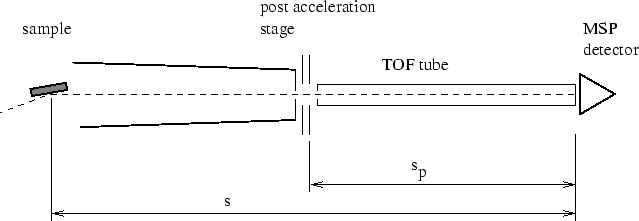To convert the time of flight spectra to energy spectra the following procedure was used [13 ,26 ,14 ]. The time-of-flight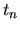for a neutral particle scattered from the sample towards the detector can be calculated as follows (Figure  5.2 ):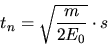(5.1)

where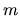is the particle mass and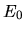the particle energy after reflection from the sample, and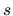is the sample - detector distance. The time-of-flight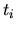of an accelerated ion is given by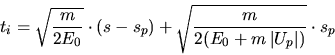(5.2)

where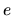is the elementary charge,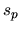the length of the tube at postacceleration potential and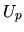the postacceleration voltage. The TOF system was calibrated for each new primary beam energy and particle mass. This was done by deflecting the positive primary beam directly into the TOF tube. We then performed a first measurement without postacceleration (thus yielding a time of flight according to Equation 5.1 ) and a second one with a certain postacceleration voltage (usually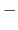2000V) giving a time of flight according to Equation 5.2 . The two time-of-flights were measured with respect to an arbitrarily chosen reference time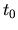, which corresponded with the start time of the particle bunch at the chopper. The difference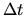of the two time-of-flights was then calculated. From the equation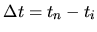the primary energywas then calculated by solving Equations 5.2 and 5.1 numerically. Using Equation 5.1 the time of flight axis could the be calibrated.

As we never observed molecules in the reflected beam all peaks in the TOF spectra are from atomic species and could be separated easily with virtually no overlap. An example spectrum showing a molecular peak species in the TOF is shown in Figure 5.6 (taken from [27 ]). For each different particle mass and charge state the corresponding rates in the TOF spectra were converted to an energy spectrum using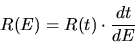(5.3)

where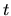and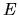denote time and energy and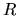the count rate in energy and time space respectively, according to the definition of distribution functions. The energy spectra obtained this way were then corrected for detection efficiency.

March 2001 - Martin Wieser, Physikalisches Institut, University of Berne, Switzerland